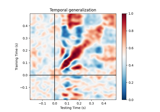# mne.decoding.cross_val_multiscore¶

mne.decoding.cross_val_multiscore(estimator, X, y=None, groups=None, scoring=None, cv=None, n_jobs=1, verbose=0, fit_params=None, pre_dispatch='2*n_jobs')[source]

Evaluate a score by cross-validation.

Parameters
estimatorinstance of sklearn.base.BaseEstimator

The object to use to fit the data. Must implement the ‘fit’ method.

Xarray_like, shape (n_samples, n_dimensional_features,)

The data to fit. Can be, for example a list, or an array at least 2d.

yarray_like, shape (n_samples, n_targets,)

The target variable to try to predict in the case of supervised learning.

groupsarray_like, with shape (n_samples,)

Group labels for the samples used while splitting the dataset into train/test set.

scoring

A string (see model evaluation documentation) or a scorer callable object / function with signature scorer(estimator, X, y). Note that when using an estimator which inherently returns multidimensional output - in particular, SlidingEstimator or GeneralizingEstimator - you should set the scorer there, not here.

cvint, cross-validation generator | iterable

Determines the cross-validation splitting strategy. Possible inputs for cv are:

• None, to use the default 3-fold cross validation,

• integer, to specify the number of folds in a (Stratified)KFold,

• An object to be used as a cross-validation generator.

• An iterable yielding train, test splits.

For integer/None inputs, if the estimator is a classifier and y is either binary or multiclass, sklearn.model_selection.StratifiedKFold is used. In all other cases, sklearn.model_selection.KFold is used.

n_jobsint

The number of jobs to run in parallel (default 1). Requires the joblib package.

verboseint, optional

The verbosity level.

fit_paramsdict, optional

Parameters to pass to the fit method of the estimator.

pre_dispatchint, or str, optional

Controls the number of jobs that get dispatched during parallel execution. Reducing this number can be useful to avoid an explosion of memory consumption when more jobs get dispatched than CPUs can process. This parameter can be:

• None, in which case all the jobs are immediately created and spawned. Use this for lightweight and fast-running jobs, to avoid delays due to on-demand spawning of the jobs

• An int, giving the exact number of total jobs that are spawned

• A string, giving an expression as a function of n_jobs, as in ‘2*n_jobs’

Returns
scoresarray of float, shape (n_splits,) | shape (n_splits, n_scores)

Array of scores of the estimator for each run of the cross validation.

## Examples using mne.decoding.cross_val_multiscore¶